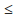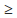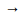# TARGET SBI EXAM 2018: REASONING – MOT-1

Dear Bankersdaily Aspirants,

Hope , Your preparation for SBI Clerk & PO exam 2018 is good . Since SBI Clerk & PO exam is a Two level which will be conducted in an online mode, Competition will be higher for this SBI Clerk & PO Exam and the most important thing , Current affairs section will be there in this exam. So aspirants have to concentrate in Four sections namely Aptitude, Reasoning, English, Current Affairs.

The person with high speed and very good accuracy only can crack this exam to taste the success. The candidates will be selected on the basis of performance in this online exam test. So the candidate with consistent hard work and regular practice will crack the exam very easily.

Exam: SBI Clerk & PO Exam 2018

Topic: MOT-1

Timing: 7 minutes

Q.1) Which of the following symbol to be used in the given equation 4_2_3_3=11 to make it as true.

a) ×, -, +

b) -, +, ÷

c) ÷, +, ÷

d) ÷, +, ×

e) None of these

Q.2) If ‘*’ means ‘multiplication’, ‘@’ means ‘division’, ‘&’ means ‘subtraction’, ‘\$’ means ‘addition’, then 10\$5*7&12*9@3=?

a) 10

b) 9

c) 11

d) 12

e) None of these

Q.3) If P>Q>R=T≤K≥D=S, then which of the given conclusion is definitely true?

a) P>T

b) R≤D

c) K≥S

d) R≤K

e) All are true except option (b)

D.4-8) In each question, statements are given, which are followed by two conclusions I & II. You have to find out which conclusion(s) is/are definitely true.

Q.4) Statement: T>Y, Y<W, W≥E, E≥I, I<S

Conclusions:

I.W>S

II.W≤S

a) if only conclusion I is true.

b) if only conclusion II is true.

c) if either conclusion I or II is true.

d) if neither conclusion I nor II is true.

e) if both conclusions I and II are true.

Q.5) Statement: Y≥S, S=F, F<G, G<Q, Q=P

Conclusions:

I. Y≥F

II.F<P

a) if only conclusion I is true.

b) if only conclusion II is true.

c) if either conclusion I or II is true.

d) if neither conclusion I nor II is true.

e) if both conclusions I and II are true.

Q.6) Statement: J=K, KF, FD, D>A, A>W

Conclusions:

I. K>W

II.K=W

a) if only conclusion I is true.

b) if only conclusion II is true.

c) if either conclusion I or II is true.

d) if neither conclusion I nor II is true.

e) if both conclusions I and II are true.

Q.7) Statement: MN, W<N, I>W, M=P

Conclusions:

I. M<I

II.N≤P

a) if only conclusion I is true.

b) if only conclusion II is true.

c) if either conclusion I or II is true.

d) if neither conclusion I nor II is true.

e) if both conclusions I and II are true.

Q.8) Statement: LP, R<L, P=J, J>D

Conclusions:

I. L≥J

II.R>D

a) if only conclusion I is true.

b) if only conclusion II is true.

c) if either conclusion I or II is true.

d) if neither conclusion I nor II is true.

e) if both conclusions I and II are true.

D.9-10) In the following questions, the symbols %, \$, @, &, # are used with the following meanings as illustrated below:

P % Q’ means ‘P is greater than Q’

P \$ Q’ means ‘P is greater than or equal to Q’

P @ Q’ means ‘P is smaller than Q’

P & Q’ means ‘P is less than or equal to Q’

P # Q’ means ‘P is neither less than nor greater than Q’

Now, in each of the following questions, assuming the given statements to be true, find which of the three conclusions I, II and III given below them is / are definitely true.

Q.9) Statements: Q @ W, W \$ R, R # T, T % Y, Y & P Conclusions:

I. Q \$ T

II. R % Y

III. R & P

a) Only I follows

b) Only II follows

c) Only both I and III follow

d) Only both II and III follow

e) None of these

Q.10) Statements: N @ M, M & V, V & C, C & H, H % A

Conclusions:

I. N & V

II. V & A

III. N & H

a) Only I follows

b) Only both II and III follow

c) Only both I and II follow

d) None follows

e) None of these

1. (d)

4 ÷ 2 + 3 × 3 = 11

2. (b)

*×

@÷

&\$+

10 + 5 × 7 – 12 × 9 ÷ 3

10 + 35 – 36 = 9

3. (e)

P > Q > R = T ≤ K ≥ D = S

(a) P > T (True)

(b) R ≤ D (False)

(c) K ≥ S (True)

(d) R ≤ K (True)

4. (c)

T > Y < W ≥ E ≥ I < S

I. W > S (False)

II. W ≤ S (False)

5. (e)

Y ≥ S = F < G < Q = P

I. Y ≥ F (True)

II. F < P (True)

6. (d)

J = K ≤ F ≤ D > A > W

I. K > W (False)

II. K = W (False)

7. (b)

P = M ≥ N > W < I

I. M < I (False)

II. N ≤ P (True)

8. (a)

R < L ≥ P = J > D

I. L ≥ J (True)

II. R > D (False)

D.9-10)

9. (b)

Q < W ≥ R = T > Y ≤ P

I. Q ≥ T (False)

II. R > Y (True)

III. R ≤ P (False)

10. (d)

N < M ≤ V ≤ C ≤ H > A

I. N ≤ C (False)

II. V ≤ A (False)

III. N ≤ H (False)

For the other Sections in the Day 3 of the TARGET SBI PLANNER , please check the below given links.

Aspirants can also check the other topics from the SBI CLERK STUDY PLANNER from the link given below. DO bookmark the given link as all the posts will be updated in that page and aspirants can check the daily updates from this page or from the Homepage of Bankersdaily.